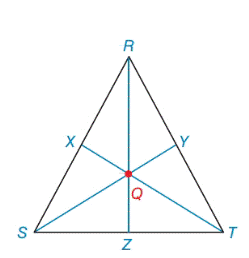Chapter 7.2, Problem 30EElementary Geometry For College St...

7th Edition
Alexander + 2 others
ISBN: 9781337614085

Solutions

Chapter
SectionElementary Geometry For College St...

7th Edition
Alexander + 2 others
ISBN: 9781337614085
Textbook Problem

Given: Isosceles Δ R S T R S = R T = 10         a n d         S T = 16. Medians R Z ¯ , T X ¯ and S Y ¯ meet at centroid Q .Find: R Q         and           Q TExercises 29, 30

To determine

To find:

The RQandQT in the given isosceles triangle RST.

Explanation

Definition:

The three medians of a triangle are congruent at a point that is two-thirds the distance from any vertex to the midpoint of the opposite side.

Formula used:

The triangle RST, with sides a,bandc opposite vertices R,SandT. Then the length of the median ma form R is given by

ma=122(b2+c2)a2

The length of the median mc form T is given by

mc=122(a2+b2)c2

Where ma,mc denotes the length of the median

Given:

In ΔRST, medians RZ¯, TX¯ and SY¯ intersect at centroid Q. If RS=RT=10andST=16.

Calculation:

The point of concurrence Q for the three medians of a triangle is known as centroid of the triangle. In ΔMNP, medians MB¯, NA¯ and PC¯ intersect at centroid Q.

According to the definition,

RQ=23(RZ)(1)QT=23(TX)(2)

Given RS=RT=10andST=16.

The triangle RST, with sides a,bandc opposite vertices R,SandT.

So, ST=16=a,RT=10=bandRS=10=c.

We sketch the figure of the isosceles triangle RST with sides a,bandc

First we need to find length of the median RZ,

Using the above formula,

The length of the median ma form R is given by

RZ=ma=122(b2+c2)a2=122(102+102)162=122(100+100)256=122(200)256=12400256=12144Ȁ

Still sussing out bartleby?

Check out a sample textbook solution.

See a sample solution

The Solution to Your Study Problems

Bartleby provides explanations to thousands of textbook problems written by our experts, many with advanced degrees!

Get Started

21. If has the graph in Problem 5(a), find the following. (a) f(9) (b) f(5)

Mathematical Applications for the Management, Life, and Social Sciences

In Exercises 3540, rationalize the numerator of each expression. 39. 1+x+2x+2

Applied Calculus for the Managerial, Life, and Social Sciences: A Brief Approach

Evaluate the integral. dxx2+2x+5

Calculus (MindTap Course List)

Simplify the expressions in Exercises 97106. 21/32122/321/3

Finite Mathematics and Applied Calculus (MindTap Course List)

The length of the curve given by x = 3t2 + 2, y = 2t3, is:

Study Guide for Stewart's Multivariable Calculus, 8th